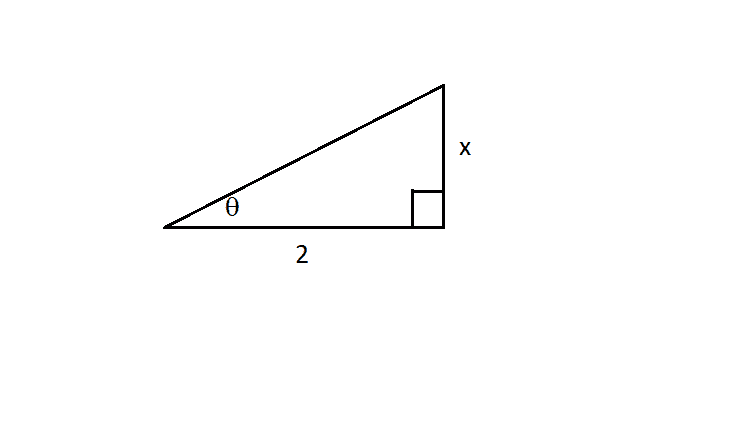# Integrate ##\int\sqrt{4+x^2} dx##

• chwala
correct! i just checked...i ended up with,$$\int \sqrt{(8-4\sinh^2 u)}⋅ 2 \sinh u du$$...not looking good!

#### chwala

Gold Member
Homework Statement
$$\int \sqrt{4+x^2} dx$$
Relevant Equations
hyperbolic equations
still typing...checking latex

This is a textbook question...the steps to solution are pretty easy...they indicated use of;

##x=2\sinh u## that will realize

$$\int \sqrt{4+x^2} dx=\int 2\cosh u⋅ 2\cosh u du=4\cosh^2 u du$$

...from here the steps are pretty clear up to the required solution.

Ok to my question now; Could we also use;

##x=2\cosh u## instead of ##x=2\sinh u##?

Cheers

Last edited:
chwala said:
Ok to my question now; Could we also use;

##x=2\cosh u## instead of ##x=2\sinh u##?

Cheers
That doesn't look so promising to me.

PeroK said:
That doesn't look so promising to me.
I thought it will be other way round...let me try and see what comes out of it...will share later. Cheers mate.

chwala said:
Ok to my question now; Could we also use;

##x=2\cosh u## instead of ##x=2\sinh u##?
No. The point is to rewrite ##\sqrt{4 + x^2} = \sqrt{4(1+\sinh^2(u))} = 2 \cosh(u)## using the hyperbolic one. If you would have attempted to use ##x = 2 \cosh(u)## instead you would have ended up with ##\sqrt{4 + x^2} = 2\sqrt{1+\cosh^2(u)}## and ##1 + \cosh^2(u)## does not have any particular simplification in terms of the hyperbolic one. If you would attempt to replace ##\cosh^2(u)## by ##\sinh^2(u)## using the hyperbolic one you would instead end up with ##2 + \sinh^2(u)##, which doesn't make you any happier.

•chwala
Another alternative is to use the substitution ##\tan(\theta) = \frac x 2##. This is based on drawing a right triangle with an acute angle ##\theta##, where the base is 2 and the side opposite is ##x##.

With this substitution, the integral ##\int \sqrt{x^2 + 4}~dx## becomes ##4\int \sec^3(\theta)~d\theta##, an integral so well-known there's a wikipedia article devoted to it.

•WWGD and chwala
Mark44 said:
Another alternative is to use the substitution ##\tan(\theta) = \frac x 2##. This is based on drawing a right triangle with an acute angle ##\theta##, where the base is 2 and the side opposite is ##x##.

With this substitution, the integral ##\int \sqrt{x^2 + 4}~dx## becomes ##4\int \sec^3(\theta)~d\theta##, an integral so well-known there's a wikipedia article devoted to it.
Thanks @Mark44 Let me study on this approach...

The picture I was describing looks like this. The hypotenuse is ##\sqrt{x^2 + 4}##.•WWGD and chwala
Its a good rule of thumb to consider trigonometric substitutions when seeing the likes of ## \sqrt {a^2 \pm x^2}dx ##
When it's a +, consider secant, tangent;
when it's a - , consider sin, cos
Subbing ##u=ax## in each case, as
##1 \pm x^2## is " translatsble" to either of these.
Edit: Of course, consider the issue of limits of integration.

Last edited:
•chwala
Orodruin said:
No. The point is to rewrite ##\sqrt{4 + x^2} = \sqrt{4(1+\sinh^2(u))} = 2 \cosh(u)## using the hyperbolic one. If you would have attempted to use ##x = 2 \cosh(u)## instead you would have ended up with ##\sqrt{4 + x^2} = 2\sqrt{1+\cosh^2(u)}## and ##1 + \cosh^2(u)## does not have any particular simplification in terms of the hyperbolic one. If you would attempt to replace ##\cosh^2(u)## by ##\sinh^2(u)## using the hyperbolic one you would instead end up with ##2 + \sinh^2(u)##, which doesn't make you any happier.
correct! i just checked...i ended up with,

$$\int \sqrt{(8-4\sinh^2 u)}⋅ 2 \sinh u du$$...

not looking good!

Last edited: